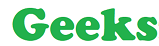# Python PIL – Image.crop() method

## Python PIL | Image.crop() method

PIL is the Python Imaging Library which provides the python interpreter with image editing capabilities. PIL.Image.crop() method is used to crop a rectangular portion of any image.

Syntax: PIL.Image.crop(box = None)
Parameters:
box – a 4-tuple defining the left, upper, right, and lower pixel coordinate.
Return type: Image (Returns a rectangular region as (left, upper, right, lower)-tuple).
Return: An Image object.

Code #1:

 `# Importing Image class from PIL module` `from` `PIL ``import` `Image` `# Opens a image in RGB mode` `im ``=` `Image.``open``(r``"C:\Users\Admin\Pictures\geeks.png"``)` `# Size of the image in pixels (size of original image)` `# (This is not mandatory)` `width, height ``=` `im.size` `# Setting the points for cropped image` `left ``=` `5` `top ``=` `height ``/` `4` `right ``=` `164` `bottom ``=` `3` `*` `height ``/` `4` `# Cropped image of above dimension` `# (It will not change original image)` `im1 ``=` `im.crop((left, top, right, bottom))` `# Shows the image in image viewer` `im1.show()`

Original ImageOutput:Code #2:

 `# Importing Image class from PIL module` `from` `PIL ``import` `Image` `# Opens a image in RGB mode` `im ``=` `Image.``open``(r``"C:\Users\Admin\Pictures\network.png"``)` `# Setting the points for cropped image` `left ``=` `155` `top ``=` `65` `right ``=` `360` `bottom ``=` `270` `# Cropped image of above dimension` `# (It will not change original image)` `im1 ``=` `im.crop((left, top, right, bottom))` `# Shows the image in image viewer` `im1.show()`

Original Image:Output:Last Updated on October 28, 2021 by admin

## Python | Pandas dataframe.round()Python | Pandas dataframe.round()

Pandas dataframe.round()   Pandas dataframe.round() function is used to round a DataFrame to a variable

## Python Input Methods for Competitive ProgrammingPython Input Methods for Competitive Programming

Python Input Methods for Competitive Programming Python is an amazingly user-friendly language with the only

## Python program to find smallest number in a listPython program to find smallest number in a list

Given a list of numbers, the task is to write a Python program to find

## Python OpenCV – cv2.rotate() methodPython OpenCV – cv2.rotate() method

Python OpenCV – cv2.rotate() method OpenCV-Python is a library of Python bindings designed to solve computer

## Reset Index in Pandas DataframeReset Index in Pandas Dataframe

Reset Index in Pandas Dataframe   Let’s discuss how to reset index in Pandas DataFrame.

## Python program to convert decimal to binary numberPython program to convert decimal to binary number

Given a decimal number as input, the task is to write a Python program to

## Pandas dataframe groupby()Pandas dataframe groupby()

Python – Pandas dataframe groupby()   Pandas groupby is used for grouping the data according

## Python – Output FormattingPython – Output Formatting

Python – Output Formatting There are several ways to present the output of a program,